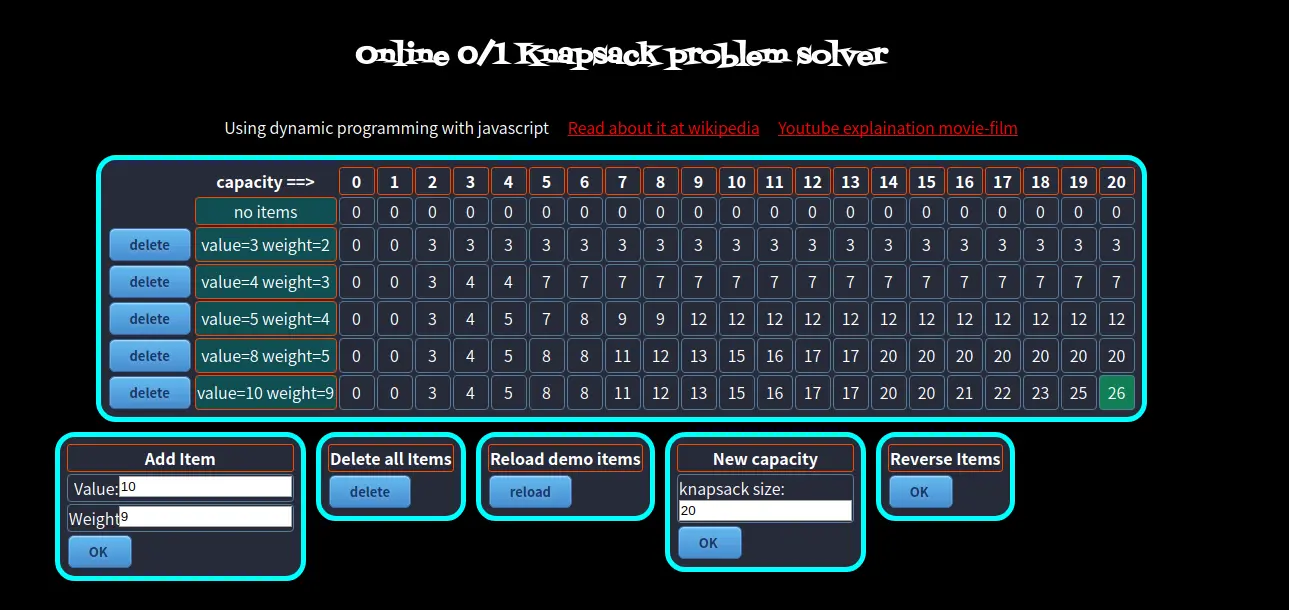## 正文

1 2 3
2 3 4
3 4 5
4 5 8
5 9 10

k 表示你面对的物品编号，即 1~5, c 表示你面对 k 号物品时，背包的剩余容量 b(k,c)表示面对 k 号物品，并作出拿或不拿的选择之后，背包里面的物品总价值

1. 如果第 k 件物品的重量 w[k]比此时的背包的剩余重量 c 大了，那我肯定是拿不动了，即`w[k]>c`。所以此时包中物品的价值就是我拿的前一个物品之后包中的价值，即 `b(k,c)=b(k-1,c).`包中剩余空间不变，还是 c。

1. 不拿 k 号物品，那么此时包中物品的总价值`b(k,c)=b(k-1,c)`，和第一种拿不动 k 号物品的一样。
2. 拿走 k 号物品，那么此时包中物品的总价值`b(k,c)=b(k-1,c-w[k])+v[k]`拿了第 k 件物品后，那我的包中的价值肯定就是原先的价值再加上第 k 件物品的价值，而且拿了之后包中的剩余容量就为`c-w[k]`了。 总结一下，就是如下的公式了：`b(k,c)=max{b(k-1,c),b(k-1,c-w[k])+v[k]}`

``````class Main {
public static void main(String[] args) {
int[] w = { 0, 2, 3, 4, 5, 9 };
int[] v = { 0, 3, 4, 5, 8, 10 };
int N = 6, W = 21;
int[][] b = new int[N][W];
for (int k = 1; k < N; k++) {
for (int c = 1; c < W; c++) {
if (w[k] > c) {
b[k][c] = b[k - 1][c];
} else {
int value1 = b[k - 1][c - w[k]] + v[k]; // 拿第k件物品
int value2 = b[k - 1][c]; // 不拿第k件物品
b[k][c] = Math.max(value1, value2);
}
}
}
System.out.println(b);
}
}
``````

## 推荐

1. 推荐一个良心视频，讲解的也很详细——01 背包问题
2. 推荐一个在线查看解决“01 背包问题”的网站，详细的描述了变化过程Online 0/1 Knapsack problem solver